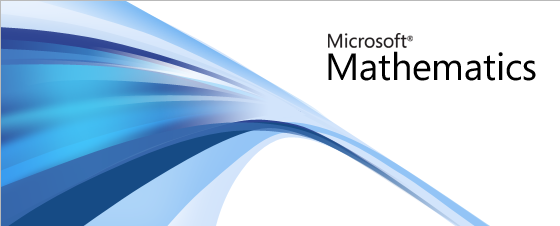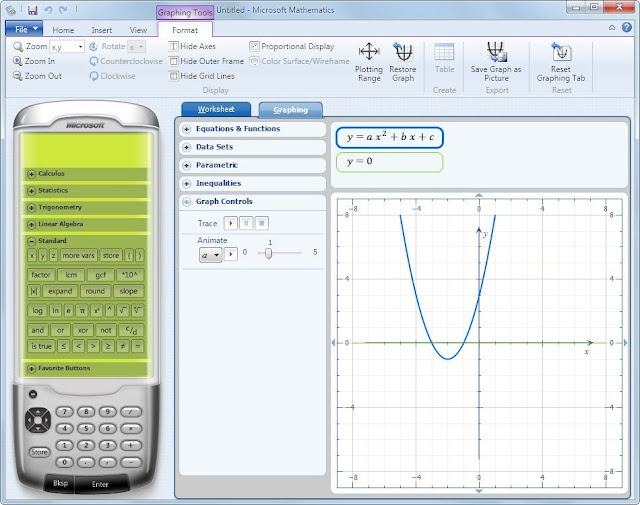Microsoft Mathematics provides a graphing calculator that plots in 2D and 3D, step-by-step equation solving, and useful tools to help students with math and science studies.Microsoft Mathematics provides a set of mathematical tools that help students get school work done quickly and easily. With Microsoft Mathematics, students can learn to solve equations step-by-step while gaining a better understanding of fundamental concepts in pre-algebra, algebra, trigonometry, physics, chemistry, and calculus.

Microsoft Mathematics includes a full-featured graphing calculator that’s designed to work just like a handheld calculator. Additional math tools help you evaluate triangles, convert from one system of units to another, and solve systems of equations.Specifications :
• It also includes a triangle solver, and an equation solver that provides step-by-step solutions to each problem.
• Allowing the user to write out problems by hand and have them recognized by Microsoft Math.
• Including graphing calculator and a unit converter to work easily.
Details :
• File Size : 18.9 MB
• Supports for Windows XP/Vista/7/8 & 10